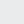# Props and Items

29978
polygons

45852
polygons

9216
polygons

--
polygons

--
polygons

28336
polygons

--
polygons

--
polygons

--
polygons

--
polygons

--
polygons

--
polygons

--
polygons

--
polygons

--
polygons

--
polygons

--
polygons

--
polygons

--
polygons

--
polygons

--
polygons

--
polygons

--
polygons

--
polygons

--
polygons

--
polygons

--
polygons

--
polygons

--
polygons

--
polygons

--
polygons

--
polygons

--
polygons

--
polygons

--
polygons

--
polygons

--
polygons

--
polygons

1024
polygons

12530
polygons

20133
polygons

3772
polygons

19812
polygons

2880
polygons

9042
polygons

2272
polygons

75428
polygons

7016
polygons

4304
polygons

## Walkie Talkie

### Props and Items

10031
polygons## Visitor## Visitor